Function Repository Resource:

# MeijerGToHypergeometricPFQ

Express MeijerG expressions in terms of HypergeometricPFQ

Contributed by: Jan Mangaldan
 ResourceFunction["MeijerGToHypergeometricPFQ"][expr] converts MeijerG expressions in expr to a form involving HypergeometricPFQ. ResourceFunction["MeijerGToHypergeometricPFQ"][expr,f] wraps f around any HypergeometricPFQ head in the result.

## Details

ResourceFunction["MeijerGToHypergeometricPFQ"] uses Slater's theorem to expand out the Meijer G-function to a sum of generalized hypergeometric functions.

## Examples

### Basic Examples (3)

A Meijer G-function:

 In:=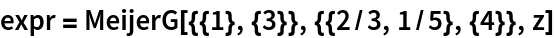Out=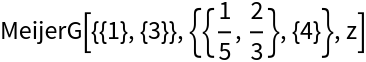Expand in terms of generalized hypergeometric functions:

 In:=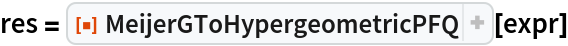Out=Verify that the two expressions are equivalent:

 In:=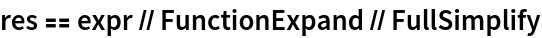Out=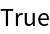### Scope (2)

A generalized Meijer G-function:

 In:=Out=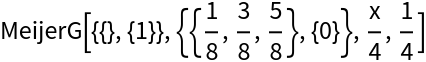Generate the hypergeometric representation:

 In:=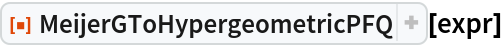Out=Use MeijerGReduce to convert a special function to its equivalent MeijerG representation:

 In:=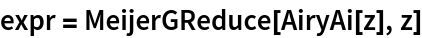Out=The result after conversion with MeijerGToHypergeometricPFQ does not have HypergeometricPFQ terms due to autoevaluation:

 In:=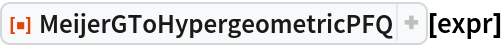Out=Wrap the HypergeometricPFQ heads in Inactive to prevent evaluation:

 In:=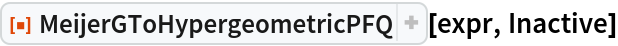Out=### Properties and Relations (3)

FunctionExpand always tries to convert to simpler functions whenever possible:

 In:=Out=MeijerGToHypergeometricPFQ performs a less drastic conversion:

 In:=Out=If Inactive is not supplied as the second argument, the resulting HypergeometricPFQ expressions might still autoevaluate:

 In:=Out=### Possible Issues (3)

MeijerG expressions that autoevaluate cannot be directly converted:

 In:=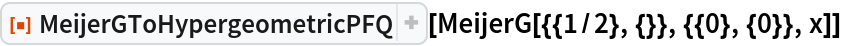Out=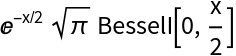In:=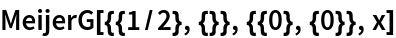Out=Wrap MeijerG in Inactive and use Inactive as the second argument to prevent evaluation:

 In:=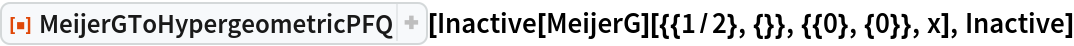Out=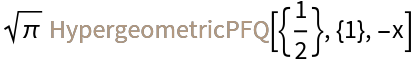Use Activate to see the simpler expression:

 In:=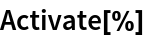Out=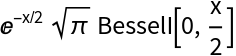Piecewise results can be returned in some cases:

 In:=Out=Logarithmic cases will not be converted:

 In:=Out=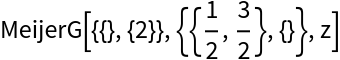## Version History

• 1.0.0 – 23 March 2021